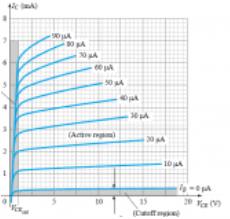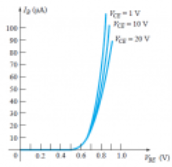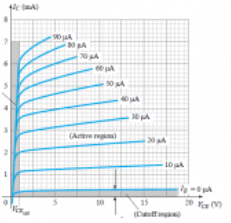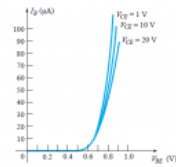Courses

# Test: BJT Configuration

## 10 Questions MCQ Test Analog Circuits | Test: BJT Configuration

Description
This mock test of Test: BJT Configuration for Electronics and Communication Engineering (ECE) helps you for every Electronics and Communication Engineering (ECE) entrance exam. This contains 10 Multiple Choice Questions for Electronics and Communication Engineering (ECE) Test: BJT Configuration (mcq) to study with solutions a complete question bank. The solved questions answers in this Test: BJT Configuration quiz give you a good mix of easy questions and tough questions. Electronics and Communication Engineering (ECE) students definitely take this Test: BJT Configuration exercise for a better result in the exam. You can find other Test: BJT Configuration extra questions, long questions & short questions for Electronics and Communication Engineering (ECE) on EduRev as well by searching above.
QUESTION: 1

### For a BJT, for common base configuration the input characteristics is represented by a plot between which of the following parameters?

Solution:

For a BJT, the input characteristics is given by a plot between VBE and IB.

QUESTION: 2

### For a BJT, for common base configuration the output characteristics is represented by a plot between which of the following parameters?

Solution:

For a BJT, the output characteristics is given by a plot between VCE and IC.

QUESTION: 3

### In a BJT, if the collector-base junction is reverse-biased and the base-emitter junction is forward-biased, which region is the BJT operating in?

Solution:

If the collector-base junction is reverse-biased and the base-emitter junction is forward-biased,then the BJT functions in the active region of the output characteristics.

QUESTION: 4

In a BJT, if the collector-base junction is forward-biased and the base-emitter junction is forward-biased, which region is the BJT operating in?

Solution:

If the collector-base junction and the base-emitter junction are both forward-biased,then the BJT functions in the saturation region of the output characteristics.

QUESTION: 5

In a BJT, if the collector-base junction and the base-emitter junction are both reverse-biased, which region is the BJT operating in?

Solution:

If the collector-base junction and the base-emitter junction are both reverse-biased,then the BJT functions in the cutoff region of the output characteristics.

QUESTION: 6

From the given characteristics, what is the value of IC at IB=30 uA and VCE=10 V?Solution:

At the intersection of IB=30 uA and VCE=10 V, IC=3.4 mA.

QUESTION: 7

From the given characteristics, what is the value of IC at VBE=0.7 V and VCE=15 V?Solution:

From the characteristics, the value of IB at VBE=0.7V is 20uA. Now, from IB=20 uA, we get IC = 2.5 mA.

QUESTION: 8

Which of the following correctly determines the relation between α and β?

Solution:

α and β are related as β=α/(1-α).

QUESTION: 9

The value of IC is precisely zero when the value of IE is zero.

Solution:

When the value of IE is zero, then the value of IC is equal to ICBO which is in the order of microamperes but not zero.

QUESTION: 10

For common emitter configuration, which of the following is the correct relation?

Solution:

All the relations hold true i.e. IC = βIB and IC = αIE. As α<1, hence IC < IE